# Reasoning Quiz For RRB NTPC : 4th January 2020

Q1. A man walked 9 km towards East and then 12 km towards South. How far is he from the starting point?
(a) 8 km
(b) 6 km
(c) 15 km
(d) 7.5 km

Directions (2-4): Select the related word/letters/number from the given alternatives.

Q2. Flow : River : : Stagnant : ?
(a) Pond
(b) Rain
(c) Stream
(d) Canal

Q3. AEIM : BFJN : : CGKO : ?
(a) DHLP
(b) ZVRP
(c) BCDK
(d) MPQR

Q4. 24 : 60 : : 210 : ?
(a) 348
(b) 336
(c) 340
(d) 326

Q5. If GOODNESS is coded as HNPCODTR then GREATNESS will be coded as:
(a) HQZFBMFRT
(b) HPFZUMERT
(c) HQEZUMFTR
(d) HQFZUMFRT

Q6. How many cubes are there in the diagram?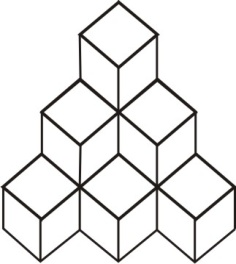(a) 10
(b) 12
(c) 8
(d) 6

Q7. Identify the diagram that best represents the relationship among classes given below
North America, United States of America, New York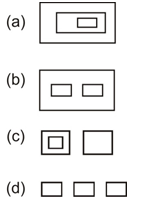Q8. Which answer figure will complete the pattern in the question figure?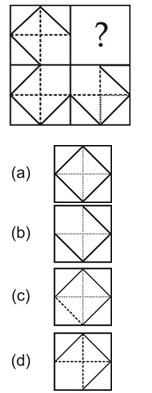Q9. A piece of paper is folded and cut as shown below in the question figures. From the given answer figures, indicate how it will appear when opened.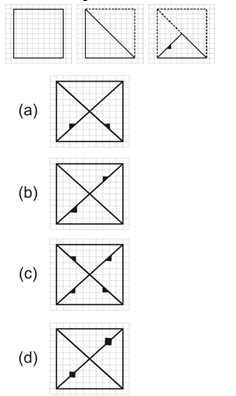Q10. If a mirror is placed on the line MN, then which of the answer figures is the right image of the given figure?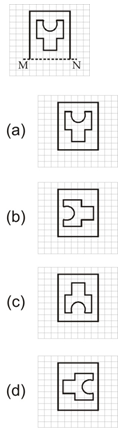### Solutions:

S1. Ans.(c)
Sol.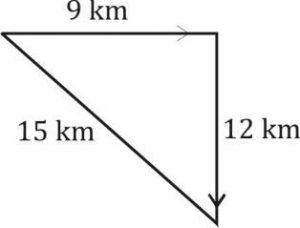→ that is the triplet of 9, 12 & 15

S2. Ans.(a)
Sol. Flow is the characteristics of river and stagnant is the characteristics of pond.

S3. Ans.(a)
Sol.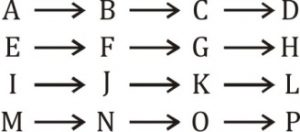but closest option is (a).

S4. Ans.(b)
Sol.
33 – 3 = 24, 73 – 7 = 336
43 – 4 =60
63 – 6 = 210

S5. Ans.(d)
Sol.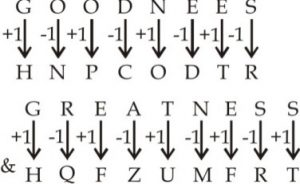S6. Ans.(a)
Sol.
The no. of cubes are in diagram-
6 + 3 + 1 = 10

S7. Ans.(a)
Sol.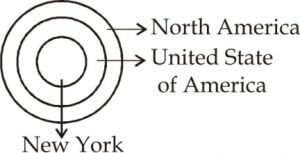S8. Ans.(d)

S9. Ans.(d)

S10. Ans.(c)

Important Links for RRB NTPC Recruitment 2019